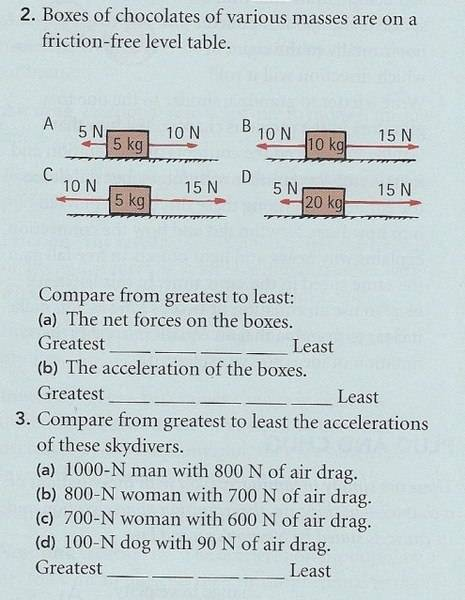# Basic Q's Net Force and Acceleration

## Homework Statement## Homework Equations

I am wondering if the answers given in the teacher's guide are incorrect.
Ans given for #2: [All same], [A,C,B,D]
Ans given for #3: [A,C,D,B]

## The Attempt at a Solution

#2a: Net forces: A,B,C are 5N; D is 10N. Therefore the order of the net forces from greatest to least is D, followed by A,B,C which are equal.
#2b: Their accelerations are a) 5N/5kg=1m/s2 b) 5N/10kg=0.5m/s2 c) 5N/5kg=1m/s2 d) 10N/20kg=0.5m/s2. Therefore the order of their accelerations from greatest to least would be A&C, which are the same, followed by B&D which are the same. Admittedly, the teacher's guide could be saying the same thing for this second part of the answer, but I can't be certain since their answer says nothing about A=C and B=D.
#3: Accelerations are:
a) a=(1000N-800N)/(1000N/9.8)=1.96m/s2
b) a=1.23 m/s2
c) a=1.4 m/s2
d) a= 0.98 m/s2
Therefore the order from greatest to least is A,C,B,D

Last edited: